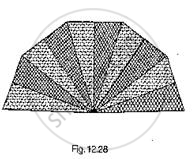# A Hand Fan is Made by Stitching Lo Equal Size Triangular Strips of Two Different Types of Paper as Shown in Fig. 12.28. - Mathematics

A hand fan is made by stitching lo equal size triangular strips of two different types of paper as shown in Fig. 12.28. The dimensions of equal strips are 25 cm, 25 cm and 14 cm. Find the area of each type of paper needed to make the hand fan.#### Solution

Given that the sides of ΔAOB are
AO = 24 cm
OB = 25 cm
BA = 14 cm
Area of each equal strips = Area of Δle AOB
Now, for area of ΔAOB
Perimeter of ΔAOB
Let 2s = AO + OB + BA

⇒s=1/2(AO+OB+BA)

=1/2(25-25-14)=32 cm

∴𝐵𝑦 𝑢𝑠𝑖𝑛𝑔 𝐻𝑒𝑟𝑜𝑛′𝑠𝑓𝑜𝑟𝑚𝑢𝑙𝑎𝑒

𝐴𝑟𝑒𝑎 𝑜𝑓 (Δ𝐴𝑂𝐵)=sqrt(s(s-ao(s-ob)s-ba))

=sqrt(32(32-25)(32-25)(32-14))

=sqrt(32(7)(4)(18))

=168 cm^2

∴ Area of each type of paper needed to make the hand fan = 5 ×(𝑎𝑟𝑒𝑎 𝑜𝑓 Δ𝐴𝑂𝐵)
= 5 × 168
= 840 cm^2

Concept: Area of a Triangle by Heron's Formula
Is there an error in this question or solution?

#### APPEARS IN

RD Sharma Mathematics for Class 9
Chapter 17 Heron’s Formula
Exercise 17.2 | Q 14 | Page 20

Share